Finding cube root by estimation

Chapter 7 Class 8 Cubes and Cube Roots
Concept wise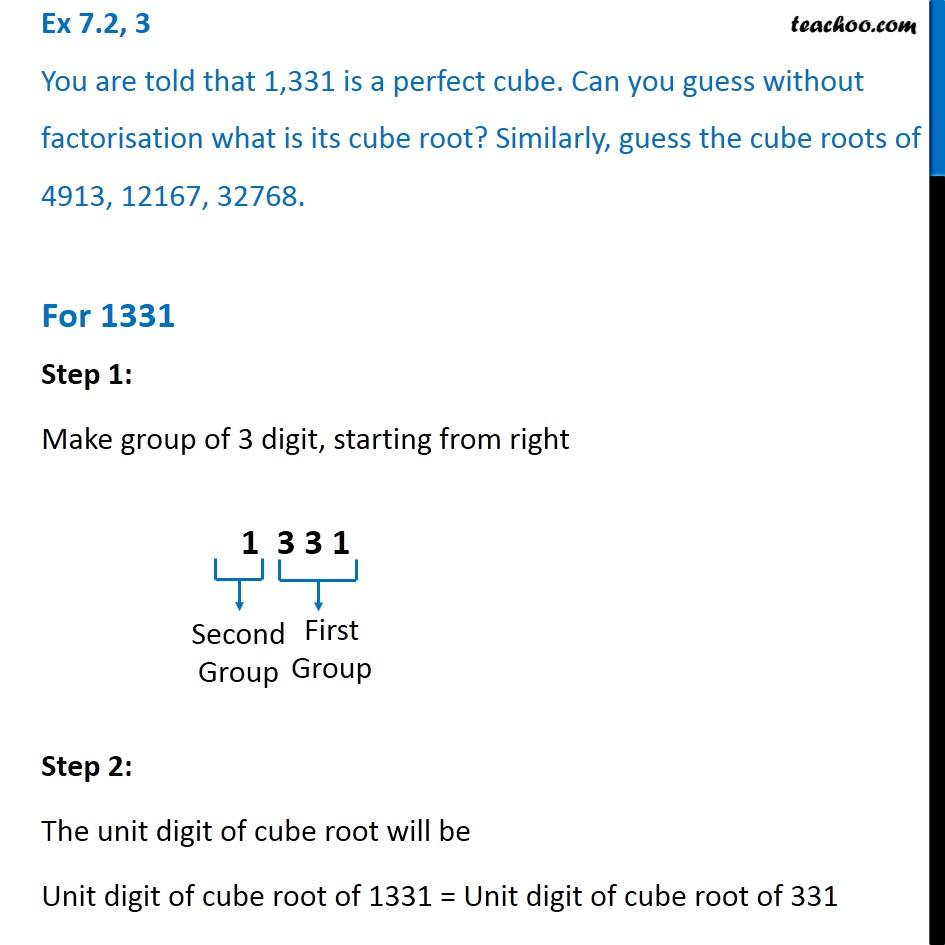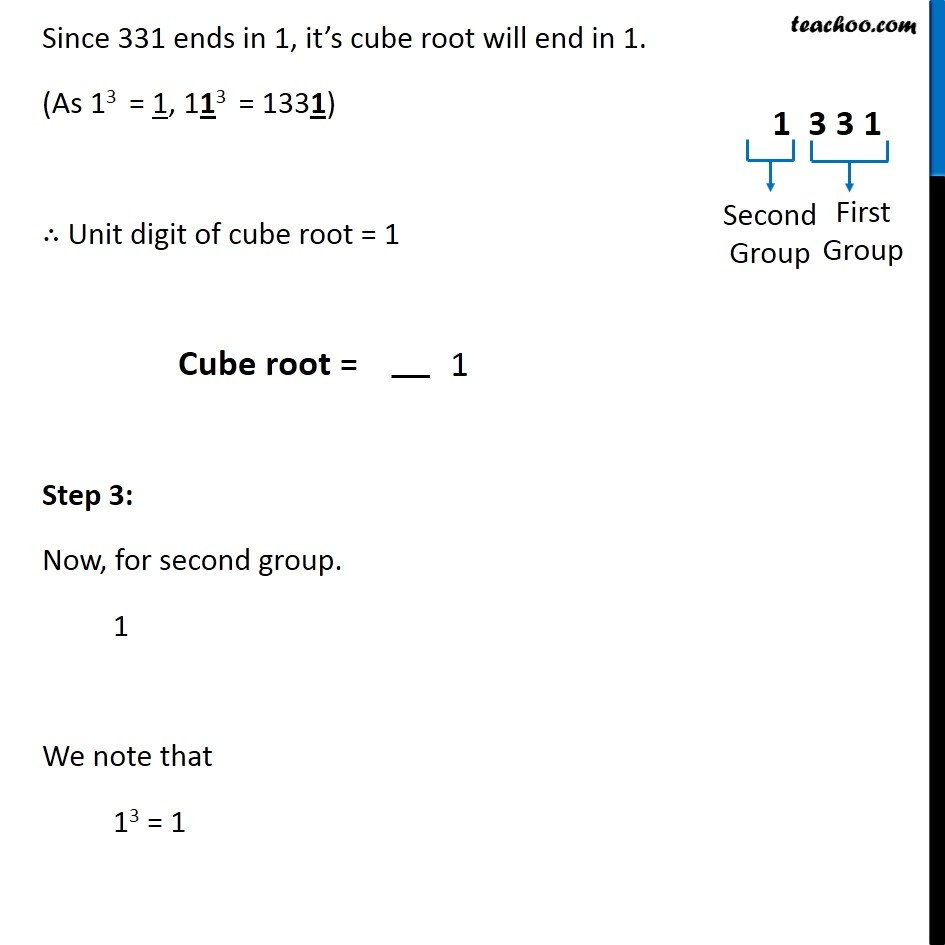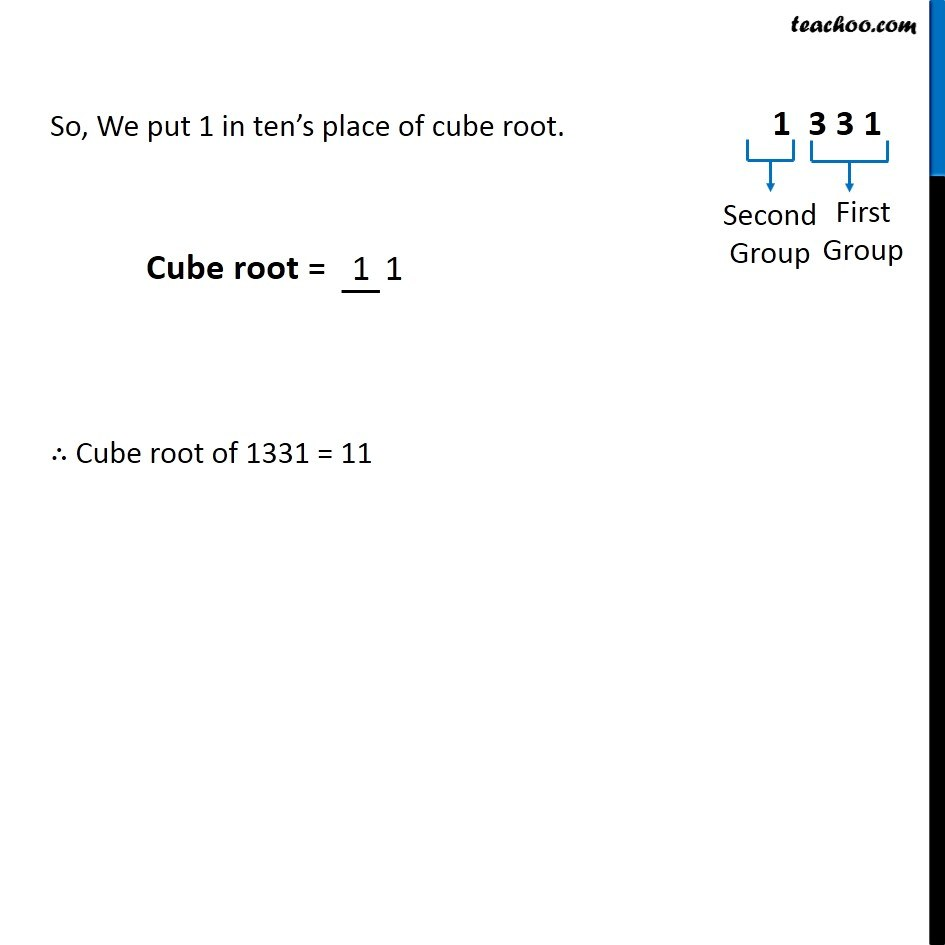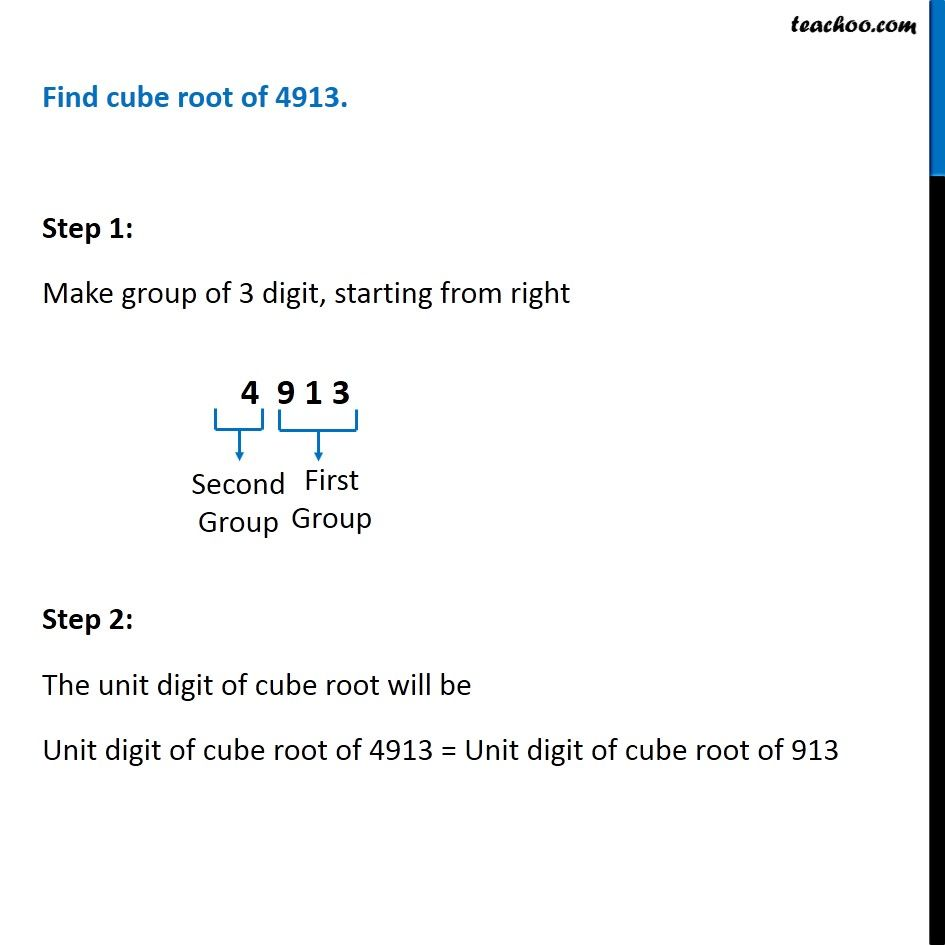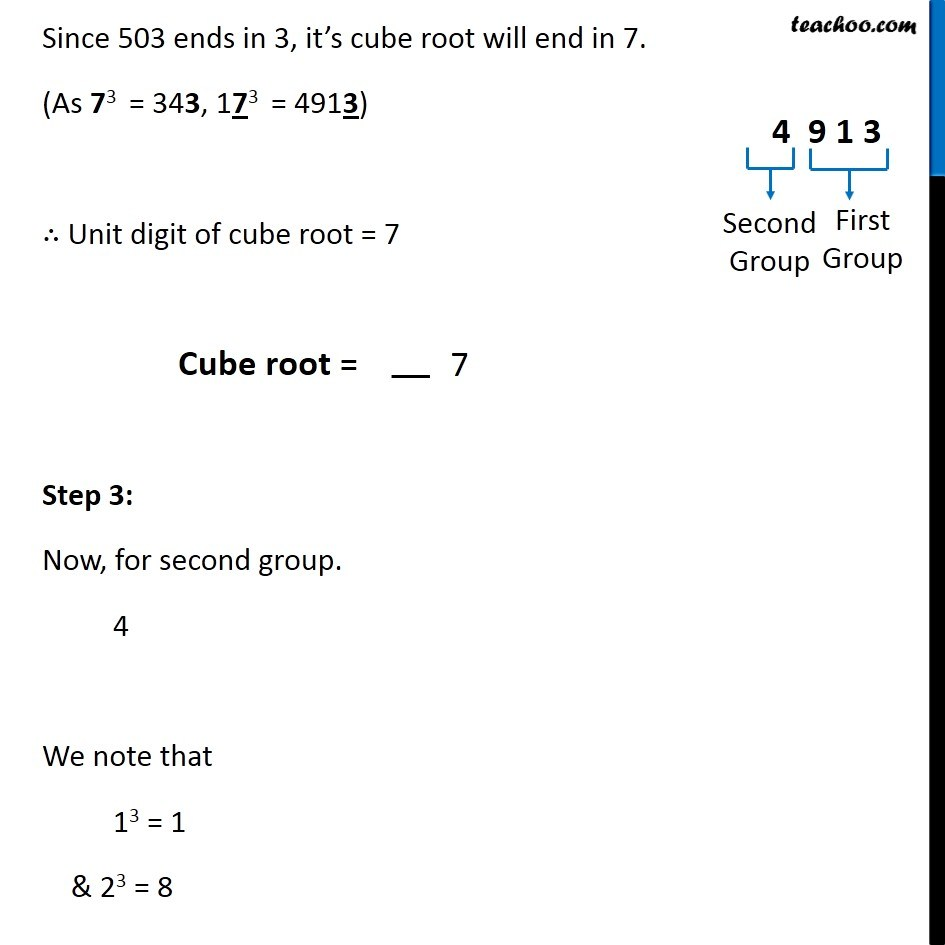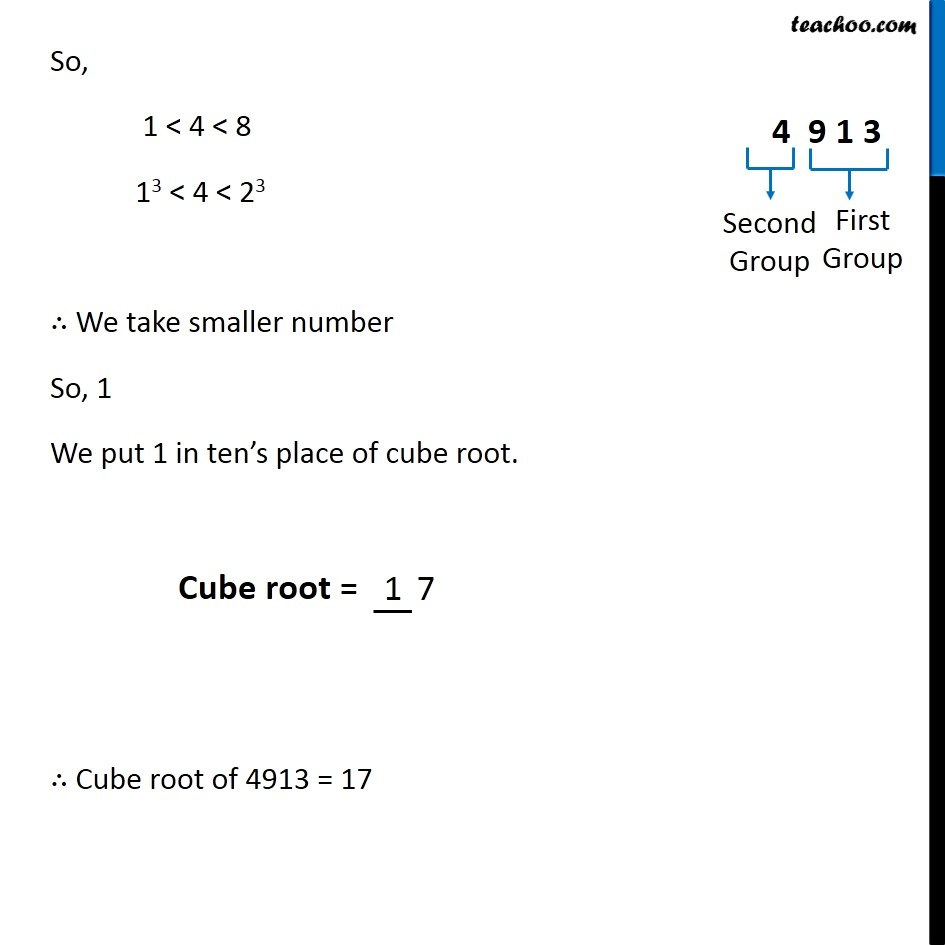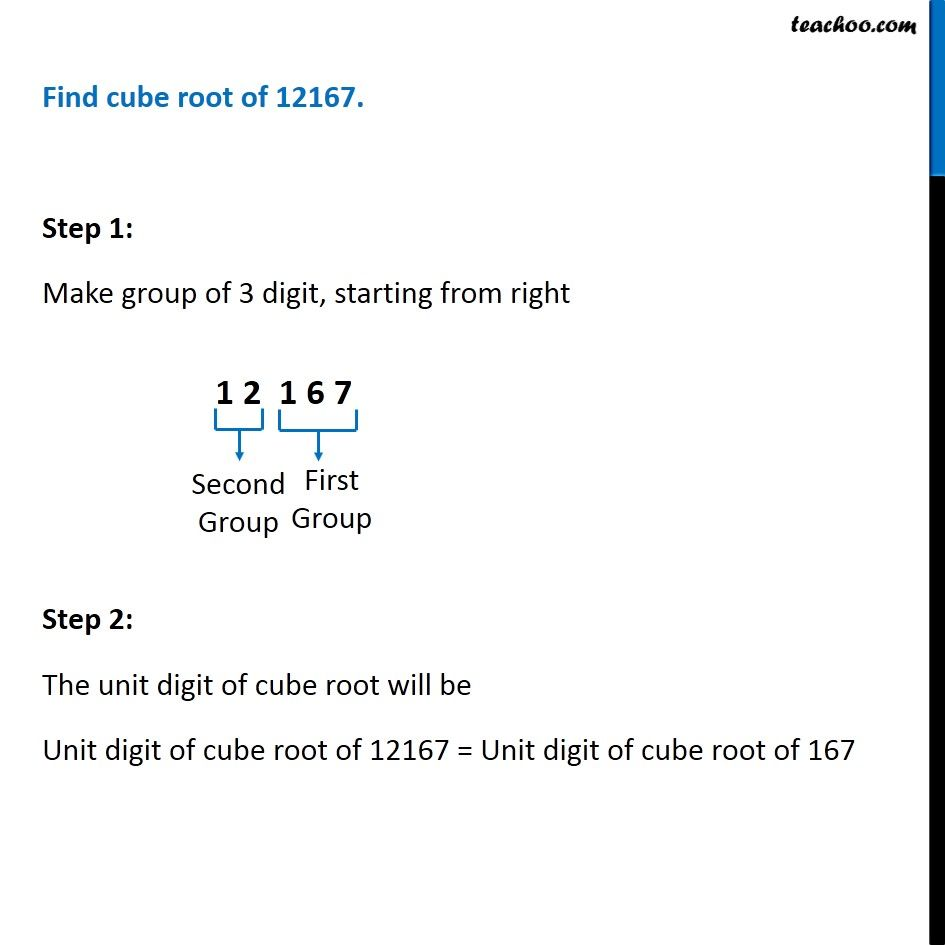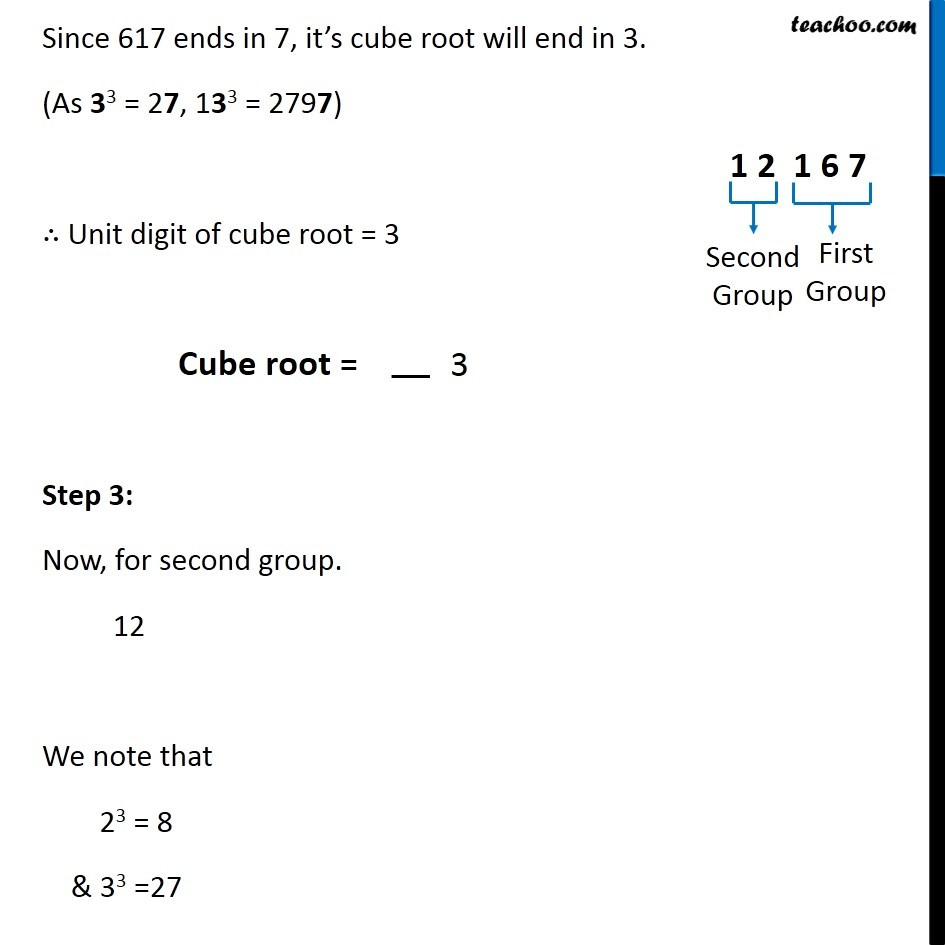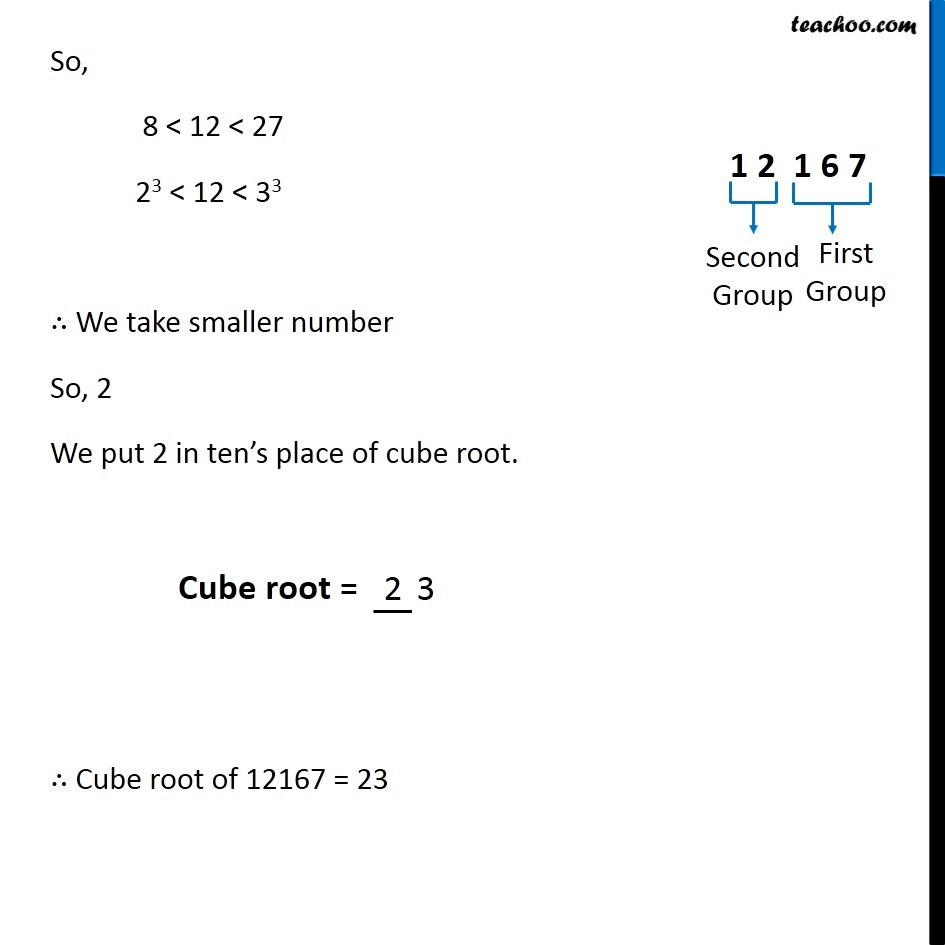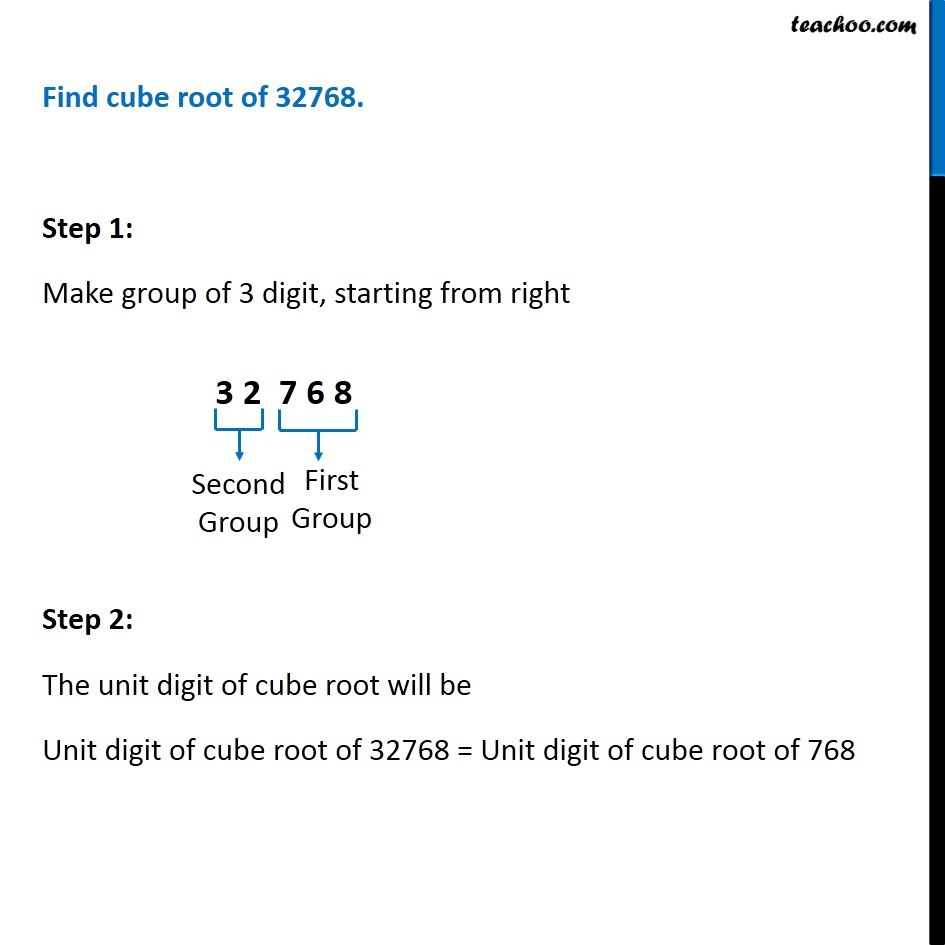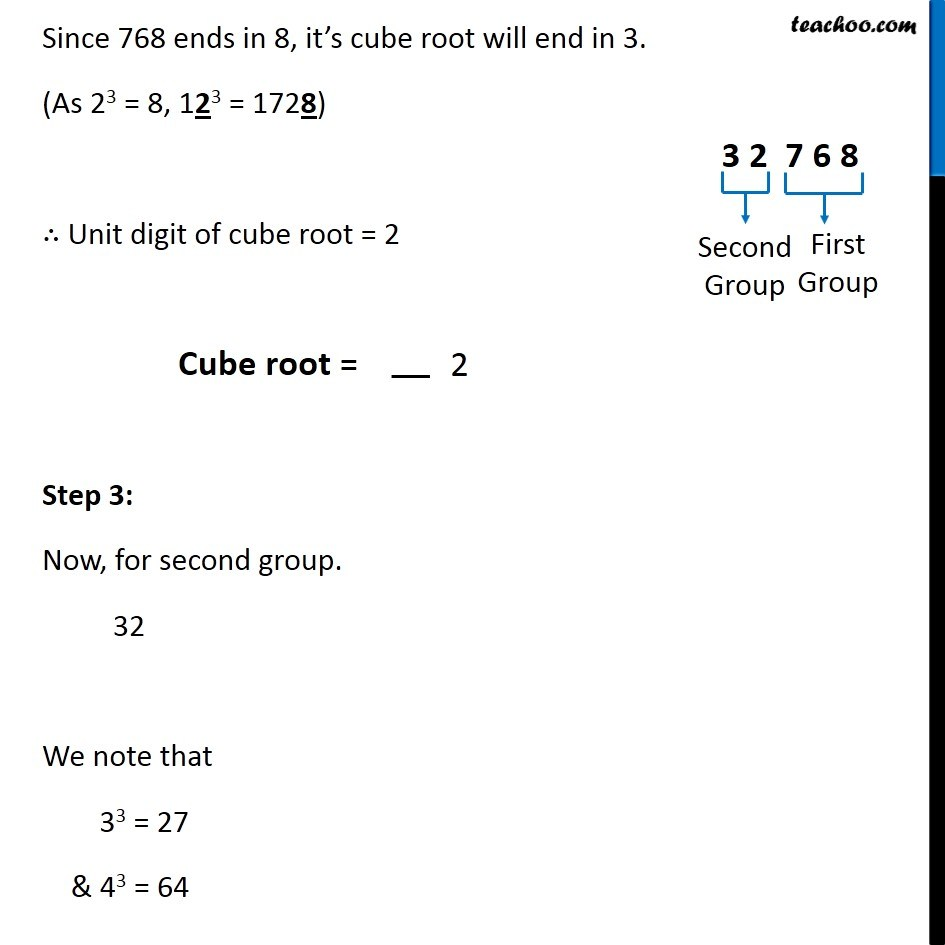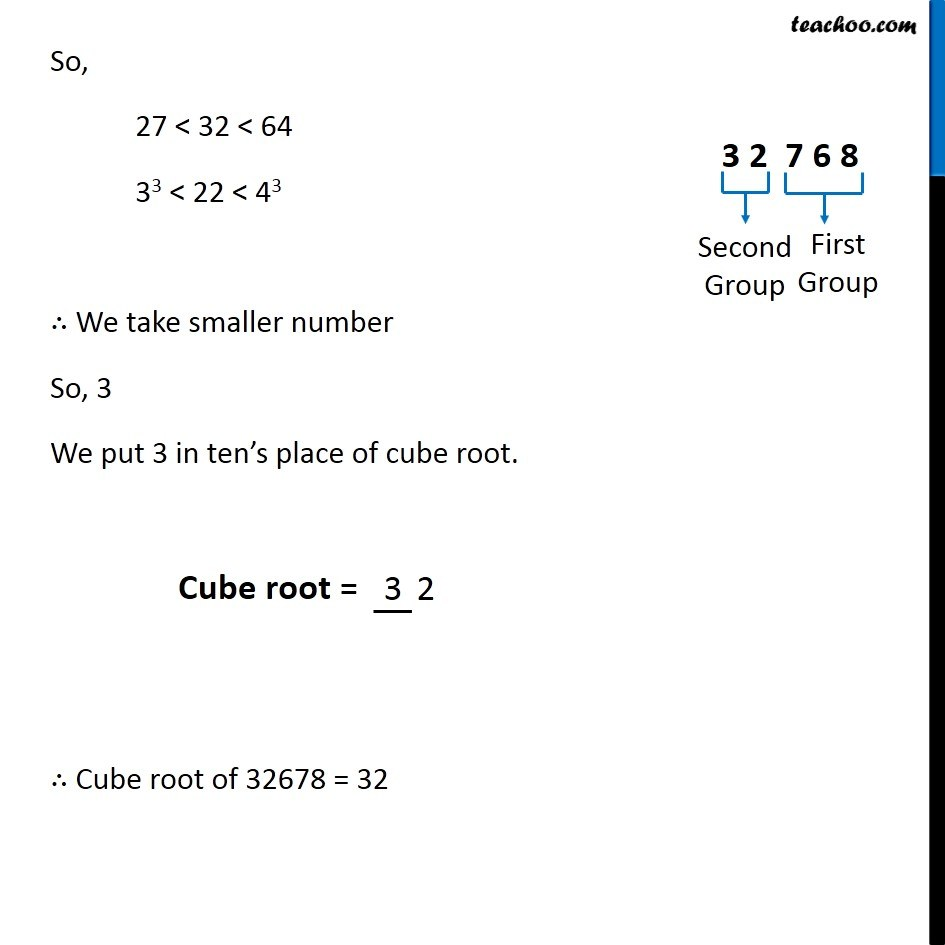Introducing your new favourite teacher - Teachoo Black, at only ₹83 per month

### Transcript

Ex 7.2, 3 You are told that 1,331 is a perfect cube. Can you guess without factorisation what is its cube root? Similarly, guess the cube roots of 4913, 12167, 32768. Step 1: Make group of 3 digit, starting from right Step 2: The unit digit of cube root will be Unit digit of cube root of 1331 = Unit digit of cube root of 331 Since 331 ends in 1, it’s cube root will end in 1. (As 13 = 1, 113 = 1331) ∴ Unit digit of cube root = 1 Step 3: Now, for second group. 1 We note that 13 = 1 So, We put 1 in ten’s place of cube root. ∴ Cube root of 1331 = 11 Find cube root of 4913. Step 1: Make group of 3 digit, starting from right Step 2: The unit digit of cube root will be Unit digit of cube root of 4913 = Unit digit of cube root of 913 Since 503 ends in 3, it’s cube root will end in 7. (As 73 = 343, 173 = 4913) ∴ Unit digit of cube root = 7 Step 3: Now, for second group. 4 We note that 13 = 1 & 23 = 8 So, 1 < 4 < 8 13 < 4 < 23 ∴ We take smaller number So, 1 We put 1 in ten’s place of cube root. ∴ Cube root of 4913 = 17 Find cube root of 12167. Step 1: Make group of 3 digit, starting from right Step 2: The unit digit of cube root will be Unit digit of cube root of 12167 = Unit digit of cube root of 167 Since 617 ends in 7, it’s cube root will end in 3. (As 33 = 27, 133 = 2797) ∴ Unit digit of cube root = 3 Step 3: Now, for second group. 12 We note that 23 = 8 & 33 =27 So, 8 < 12 < 27 23 < 12 < 33 ∴ We take smaller number So, 2 We put 2 in ten’s place of cube root. ∴ Cube root of 12167 = 23 Find cube root of 32768. Step 1: Make group of 3 digit, starting from right Step 2: The unit digit of cube root will be Unit digit of cube root of 32768 = Unit digit of cube root of 768 Since 768 ends in 8, it’s cube root will end in 3. (As 23 = 8, 123 = 1728) ∴ Unit digit of cube root = 2 Step 3: Now, for second group. 32 We note that 33 = 27 & 43 = 64 So, 27 < 32 < 64 33 < 22 < 43 ∴ We take smaller number So, 3 We put 3 in ten’s place of cube root. ∴ Cube root of 32678 = 32